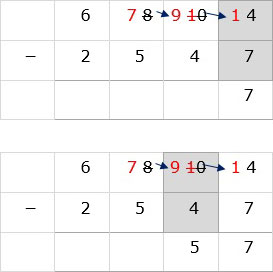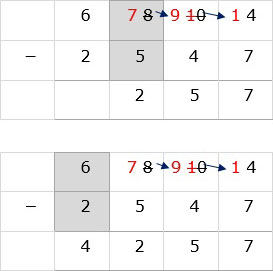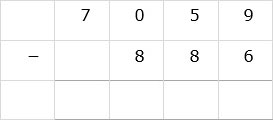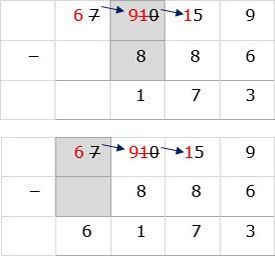# Subtraction and Regrouping with Zeros

Subtract 6804 − 2547

### Solution

Step 1:

Align the numbers vertically.Step 2:

Since 4 is less than 7, we need to regroup in the ones place. We cannot borrow from zero, so we need to regroup in tens place as well. We regroup 8 to get 7 in hundreds place and ten in tens place.Step 3:

We regroup again to get 9 in tens place and fourteen in ones place. We then subtract 7 from 14, 4 from 9, 5 from 7 and 2 from 6 to complete the subtraction.Subtract 7059 – 886

### Solution

Step 1:

Align the numbers verticallyStep 2:

In ones column subtract 6 from 9 to get 3.Step 3:

In the tens column 8 is bigger than 5, so we need to regroup and borrow.

We cannot borrow from zero in hundreds place. So we borrow from 7 in thousands place. We cross out 7 and write 6 and put 1 beside 0 to make it ten.Step 4:

To subtract 8 from 5 in tens column, we borrow from left. Cross out 10 and write 9 and put 1 beside 5 to make it 15. Then subtract 15 – 8 = 7.Step 5:

Now subtract 8 from 9 in hundreds column and bring down 6 in thousands column to get final answer as 6173.